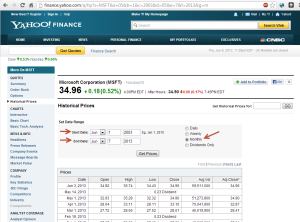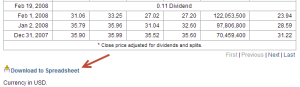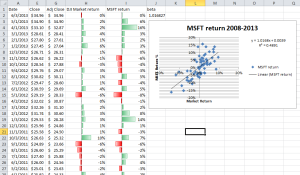# Beta analysis by period for MSFT & GOOG

Jun 06

A review of the monthly rates of return for Google and Microsoft for two successive five year periods allow me to calculate the beta, ß, for each period. Recall that ß is calculated under the Capital Asset Pricing Model (CAPM) as follows:$r-r_f = \beta(r_m-r_f)$

## Getting the data

It was easy to download the data on yahoo finance. Once there, I was able to set the time period of interest and frequency as shown here (click to enlarge).Once you have the set of data that interests you, scroll down to the bottom and download the data, as shown here.Note that in order to calculate the ß in Excel, you need the market comparison data as well. In order to get that I change my search to ^DJI with the same period and frequency as shown above. However, I noticed that they don’t provide a download option for that data. Since the data is shown in tabular format, you can copy and paste it directly in to Excel. Don’t forget to click Next until you have grabbed all the necessary pages.

Google data only went back to 2004, so the analysis for the second five year period for Google has fewer data points than the same analysis for Microsoft.

## Setup in Excel

I setup a workbook with three sheets, one for MSFT, one for GOOG and the last for DJI, or market data. Since I wanted to get two success five year periods, I added an empty line at the five year mark. I hid all but the date, closing price and adjusted closing price on each sheet.

In order to get the most accurate historical view of return rate, I used the adjusted closing price to calculate my monthly return.

#### Formatting

I then display the market data side by side with the stock specific return rate on each page. Excel conditional formatting made it easy to show movement graphically. That graphical view is a good sanity check while reviewing the formulas.

#### Scatter plot

Finally I inserted a scatter plot and used Excel’s built in linear mapping to show the ß line and calculate R-squared. Note that I also calculated ß using the SLOPE formula and provided the stock return as y and the market return as the independent variable x. This obviously agrees with the slope intercept form shown on the scatter plot.## ß stability and estimates

Here’s a summary of the data resulting from the analysis described above.

Time period Company Beta R2
2008-2013 MSFT 1.0168 0.4891
2003-2008 MSFT 0.8911 0.1381
2008-2013 GOOG 0.9526 0.3101
2003-2008 GOOG 1.2223 0.0564

As can be seen in the data, the ß was not perfectly stable between periods. However, that doesn’t mean it was drastically different. The difference is more pronounced for Google than for Microsoft.

#### Example

Let’s look at a quick example. Given a market premium of 7%, a risk free rate of 4% and using the beta from each five year period, we end up with a 2% difference between rates that should be used (see CAPM equation for ß above).

Beta period Rate r
rate ß 2008 12.556%
rate ß 2013 10.668%

The difference in r becomes more drastic as the market premium increases, but rises linearly with changes to the risk free rate.

### Conclusion

While history provides a good data point and may be used to project into the future, there are risks when using old data. When it comes to risk, there are downsides to both overestimating and underestimating. When precision like that can make the difference between dropping or executing a project, it pays to include other analysis beyond just historical review.